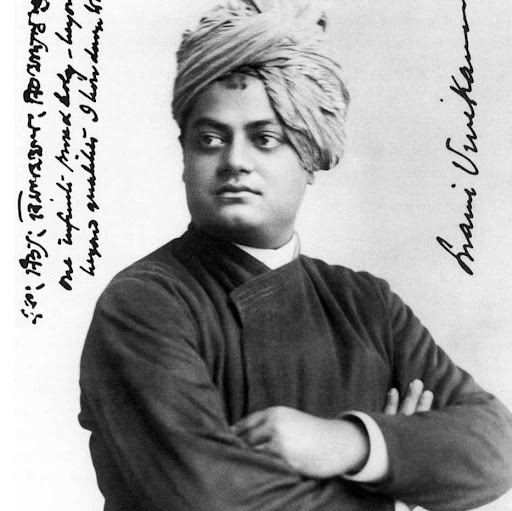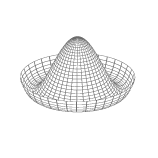1:09 AM
"billybodega"'s now-deleted answer(?) (<https://physics.stackexchange.com/users/201983/billybodega> (?)) provoked what ultimately made it to Geocast's "fun paper Friday" section this week (episode 235, <http://www.dontpanicgeocast.com/?p=898>). From 39 min 41 secs.
By Anders Sandberg. <https://arxiv.org/pdf/1807.10553.pdf>. <https://www.theatlantic.com/science/archive/2018/08/what-happens-if-the-earth-instantly-turned-in-a-giant-mass-of-blueberries/566540/>Oh sorry you can't see that on physics SEIt will take a while.

9 hours later…10:29 AM
hello

3 hours later…1:53 PM
I've got a question about associating $V^*\otimes V$ with a linear operator. If anyone has insight, I'd love to hear. It has been confusing me.
I understand the association of a linear operator $A$ with a $(1,1)$ tensor via $A(v,f) = f(Av)$, where $w,v\in V$ and $f\in V^*$. What I don't get is the association of a linear operator with $V^*\otimes V$, effectively the same space as $(1,1)$. This space maps $V\times V^*\rightarrow\mathcal{C}$, yet I see this association being done by defining a linear operator $(f\otimes v)(w) = f(w)v$.
Doesn't that entirely go against the mapping of a tensor product, instead being $V^*\otimes V:V\rightarrow V$? I understand that by expressing a $(1,1)$ tensor in the tensor product basis $T = T_i$$^je^i\otimes e_j and acting it on v via the definition above it results in T(v) = v^iT_i$$^je_j$, the appropriate expression for a linear operator, but I don't understand why that mapping makes any sense.
To summarize, it appears $(f\otimes v):V\rightarrow V$ as defined above, but it must be $V\times V^* \rightarrow \mathcal{C}$, right?
@ACuriousMind2:23 PM
@dsm What is $\mathcal{C}$?
Also what you basically have in those various tensor business is like
Partial application of a function
$f \otimes v$ can be seen as a map from $V^* \times V$ to $\mathbb{R}$, but by partial application, you also have $[f \otimes v](x, -) : V^* \to V$
it is similar to currying if you've ever encountered that
It's generally not written explicitely, though
it's implicit by the number of arguments you feed the tensor3:23 PM
@j Mac I have a question related to yesterday question.3:45 PM
@Slereah Hi there, thanks for the input. Sorry, forgot to mention that, was just denoting the set of scalars by $\mathcal{C}$. Perfect, hadn't heard of currying before, but that looks like it's exactly what's implicitly going on! So we're actually dealing with $A_w(h) \equiv \text{curry}[(f\otimes v)(w,h)] = (f\otimes v)(w)(h) = f(w)v(h)$, do I have that right? Then if we were to hit $A_w$ with some dual $h$ it would return a scalar, completing the map.3:57 PM
And now it seems like the metric dual $L:V\rightarrow V^*$ defined by $L(v)(\cdot) = (v|\cdot)$ is just the curry of the non-degenerate Hermitian form -- is that correct to say?roughly yes
instead of using a function of 2 variables to get a scalar, you input only a single variable, to obtain a function of one variable
$f : V \times V \to \mathbb{R}$ to $f : V \to (V \to \mathbb{R})$
This can work for any number of variables
if you have a function $V^n \times V^{*m}$ and apply it to $V^{n'} \times V^{*m'}$, you get a function $$V^{n - n'} \times V^{*(m - m')} \to \mathbb{R}$$
Rigorously it should be written down but in practice nobody does itOk perfect, that makes so much more sense -- thank you. Yeah, it definitely seemed like something implicitly was going on, haha wish it was even slightly mentioned. So the set $V^*\otimes V$ isn't a linear transformation, a curry of it is
Linear operator*4:19 PM
Well I mean
$V^* \otimes V$ is just a set
What you do with it is another matter~Pretty random question:
I need some common examples where the concept "motionless" is used in physics4:38 PM
@Slereah Ah, yes, I should rephrase. The curry of any element in that set is a linear transformation
Whoops, did it again... linear *operatoralso it's not quite currying
it's more of a partial applicationOhh, that seems pretty subtle, why not currying?5:49 PM
@Slereah hello :) How are you?6:06 PM
@dsm Currying is to turn functions of several variables into functions of one variableSo it's like currying without explicitly writing how that additional variable (dual vector) will play into the full mapping?6:38 PM
@dsm What do you mean when you say that the "space $V^\ast \otimes V$ maps $V \times V^\ast \to C$"?

2 hours later…8:13 PM
0Today I downvoted @Eric Amador answer What are absolute space & time? Why are they not absolute in reality? and flagged it, too. Now I get negative scores. Presumably, because I wrote a comment on a bad answer, which is downvoted by others too. Although, I do not care too much about my score, I ...